# PID, D as in Derivative

All the cheap neon letters are now lit up by your growing knowledge! We arrive at the last letter of PID: the letter D. Remember there is an introduction to PID, a proportional post for the P and an integral post for the I. It’s not the end, it’s only the beginning.

Quite a journey, it has been — and it still is. The previous posts were dealing, sometimes painfully, sometimes smoothly, with proportional and integral effects into the coded feedback loop control of an electronic system.

Today I’ll talk to you about the last effect: the derivative effect in a PID. Welcome to the D-post.

As for the previous posts, let me remind that a PID is — most of the time — a coded feedback loop control working into a system which includes, at least:

• a powered actuator (eg. a DC motor),
• a sensor (eg. a speed sensor), and,
• the associated command (most of the time an electronic board with a microcontroller).

And a reminder of what a PID is:

A PID is a tool used in an electronic device to get it to react the way it’s been programmed, while taking into account the changes of its environment and adapting to it.

The changing environment can be, for example, a dynamic charge (more or less people in an elevator), or the wind slowing the rotation of a rotating wing.

This is where I’m supposed to remind you the must-known vocabulary you will need in order to go on reading:

• Set-point: the user-defined value to be reached by the actuator (a speed, a torque, etc.).
• Process variable: the real actuator’s value, retrieved by the sensor in real time.
• Error: the difference between the set-point and the process variable.
• Steady state: when the system stops oscillating and finds its full balance.

Unlike the proportional part of a PID, the derivative part, like the integral one, can’t be used alone as it wouldn’t have any effect. Instead of that, derivative is either used along with proportional (PD), or with proportional and integral (full PID). The proportional part P is always used in a PID control.

Now, to the point:

The D stands for derivative, because it differentiates the computed error into the PID command.

Yes it does.

I explained in the last post that the computed error was a function of time ε(t), meaning that the error was continuously evolving at each instant. In mathematics, functions that are in a certain group (continuous functions) can be integrated, like we did in the I post, or in this case, differentiated.

## What is a derivative, or differentiation?

The derivative of a function is the result of differentiating this function. Right.
Let’s take a continuous function y = f(x). f is the function that transforms x into y. Easy.
Now let’s call f'(x) the derivative of f(x). f(x) has been differentiated into f'(x). All right... maybe slow down a little bit.
_Mathematically speaking, the derivative f' of f is the ratio of change in output by an infinitesimal change in input. Limits are used to show that change in the input. _Nope. Please go now.

Differentiating a function is like measuring how much the output y is changing when we make the input x change.

Hardly better.

The representation of the derivative of f at a point a would be the tangent of the f curve at this point. This tangent is a slope, and a slope is usually calculated with two points, by dividing the difference of y-axis by the difference of x-axis of these points. In this case, we imagine the two points being very close one to another in order for the slope to be the most precise possible.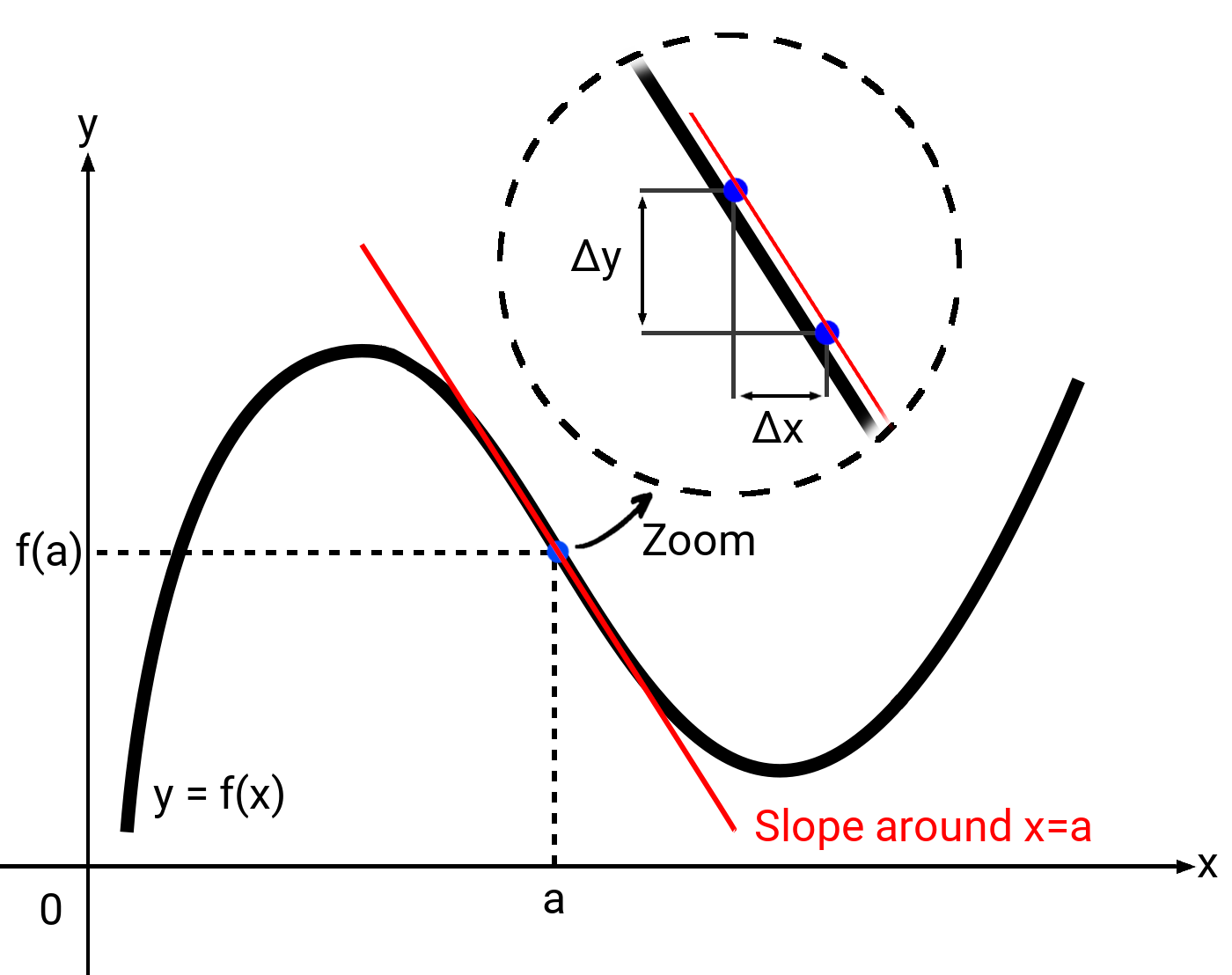So you can memorize that the derivative of a function in a point a is the slope of the curve at this point.

By the way, the notation of differentiation would be:Note: The derivative f’(x)of a function f(x) is always calculated relatively to a really small portion of its input x, which is notedΔx.
In the general notation, dx = x + Δx.

Differentiation is used every day. To take a classic example, if you differentiate the function of an object’s position with respect to time, let’s say a banana, you will obtain the velocity (speed) of this object.

Why? Because the speed is the variation of the position: the more the position changes, the more speed has your banana. What happens then if you differentiate the velocity function of a banana with respect to time? You obtain its acceleration function. Bingo. (I won’t talk to you of jerk, snap, crackle and pop, even if they actually are a thing.)

You now know what is differentiation, basically.Let’s get back to the function of the error ε(t) in the system: how to differentiate it? And why?

## Adding D to the system

The error function, as I said in the previous post, is discretized: it has a finite number of values on its curve, which are the result of each iterated loop of the system.

To add the expression fD(e) of the derivative into the command, differentiation can be simplified by subtracting the error of the previous loop to the error of the current loop, and multiply this subtraction by a factor KD (derivative gain).

Remember the extra-simplified expression of a PID? Let’s add the final bit to it.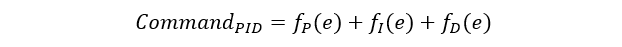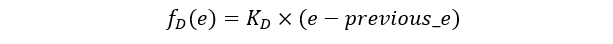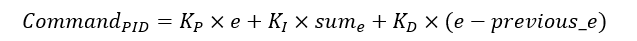e is the error, and previous_e is the error from the last loop.

Note: When you program a control loop feedback, at the very end of the actual PID loop code, the line previous_e = e is very important so that in the next passage into the loop previous_e would have a value.

Another note: We are supposed, in order to make a differentiation with respect to time, to divide e - previous_e by t - previous_t, which is the time of one loop (it depends on the sampling frequency of your system). However and most of the time, the expression of t - previous_t is implicitly included into KD.

Now it’s clear: the derivative has the effect of “predicting” what will be the error by retrieving its amount of variation, in order to reduce it.

Do you see what is my point? In other words, while integral was a “look” into the past, derivative is a “look” into the future! _(Kind of.)_Let me write it for you one more time:

The derivative looks into the future of the system.

Isn’t that sweet. But what is the real effect of this derivative element?

## The effects of D

• Derivative softens or smooths oscillations, ie. it damps the system’s response, also making it more stable.
• If correctly tuned, KD, by damping the overshoots, accelerates the global system’s response.
• But: raising KD too much will make the rise time longer and longer, because it damps the system. Also, although getting rid of overshoots and damp the whole curve seems like a good thing to do, an over-damped system will eventually show a slow response: this is not the solution you are looking for.
• In practice, many say that the gain KD is rarely used, and has good results if it is kept small.

In the following illustration, we can see the effect of the change of the derivative gain KD on the system’s response (both proportional and integral gains are held constant):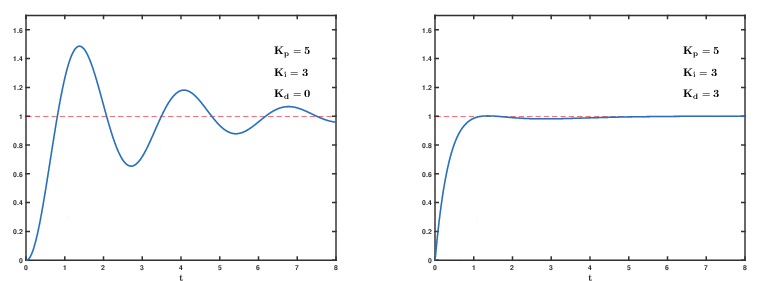Although exaggerated, this image illustrates clearly that the overshoots are damped when the derivative part is used.

Warning: the use of a derivative part works well for errors that show low dynamic changes. A temperature control, for example, is not subject to fast changes — unless your system is near a supernova, you never know.
But if the value you control is subject to very high dynamic changes or if the retrieved error is very noisy, its differentiation can result in even higher amplitude than the desired signal, leading to an unstable system.

To summarize

• The D part of a PID is the derivative command, used into a control loop feedback to control a system.
• The derivative command is used as a differentiation of the retrieved error (which is the difference between set-point and process variable), times the chosen derivative gainKD.
• Thanks to differentiation, derivative predicts what will be the error on the next loops in order to adapt the system’s response accordingly.
• Used as part of a control loop feedback, the derivative damps the curves of the system’s response.
• Derivative always comes either with a proportional command (PD), either with a proportional and an integral commands (PID) (it’s never used alone nor with an integral only).

Get Started with Luos

Tags: